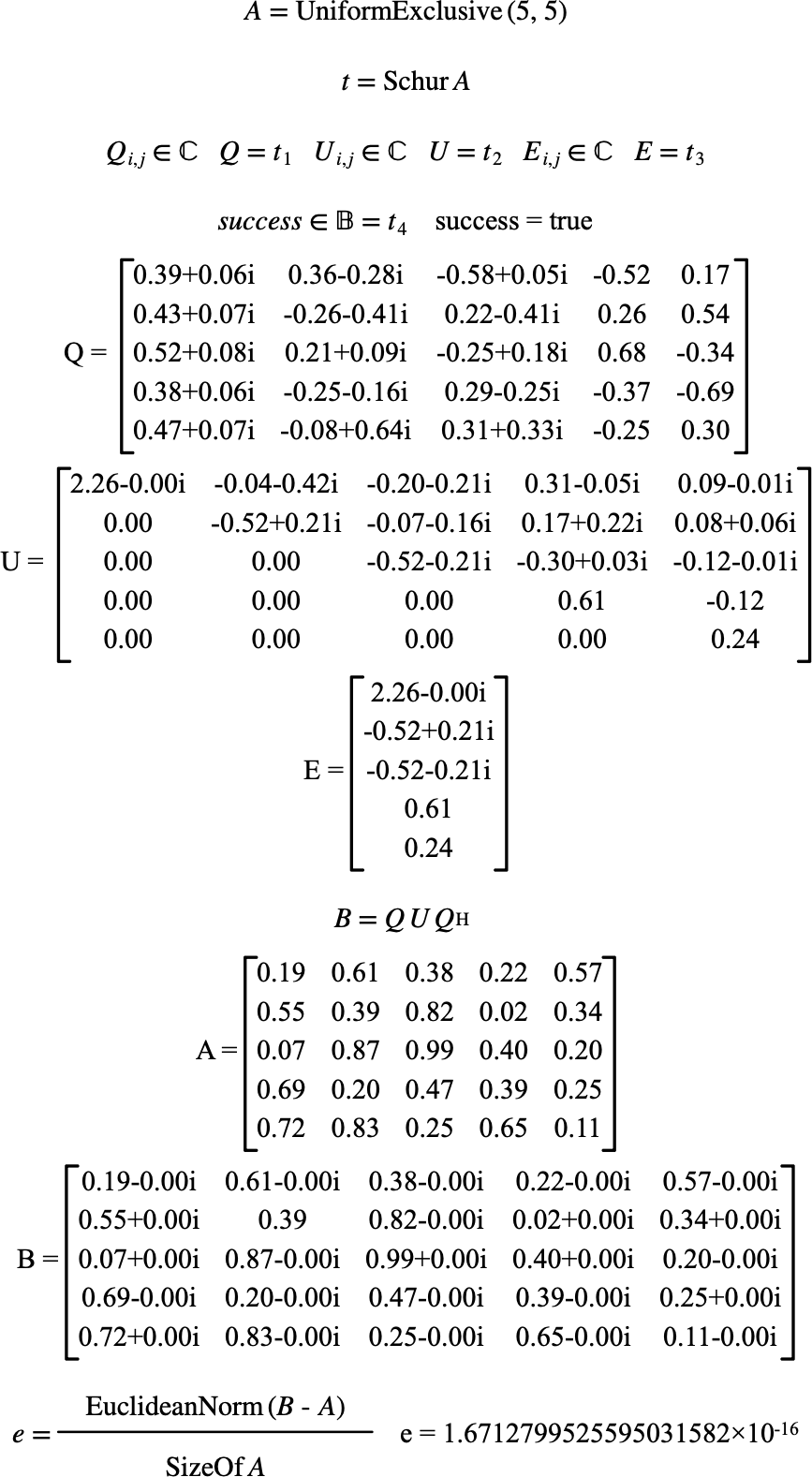# $$\text{Schur}$$¶

You can use the $$\text{Schur}$$ function to perform an Schur decomposition of a real or complex matrix, creating $$Q U Q^{-1}$$ where $$Q$$ is a unitary matrix, $$U$$ is an upper triangular matrix, and $$Q^{-1}$$ is the inverse of $$Q$$.

Performs Schur decomposition

You can use the schur backslash command to insert this function.

The following variants of this function are available:

• $$\text{tuple } \text{Schur} \left ( \text{<matrix>} \right )$$

The $$\text{<matrix>}$$ is the square matrix to calculate the Schur decomposition of.

The $$\text{Schur}$$ function returns a tuple containing:

• The complex $$Q$$ unitary matrix.

• The complex $$U$$ upper triangular matrix.

• A complex column matrix holding the diagonals of the $$U$$ matrix. This column matrix is also the eigenvalues of the supplied matrix.

• A boolean value indicating true on success or false on error.

The function is implemented internally just for complex matrices. Boolean, integer, or real matrices will be converted to a complex matrix if needed.

Figure 202 shows the basic use of the $$\text{Schur}$$ function.Figure 202 Example Use Of The Schur Function